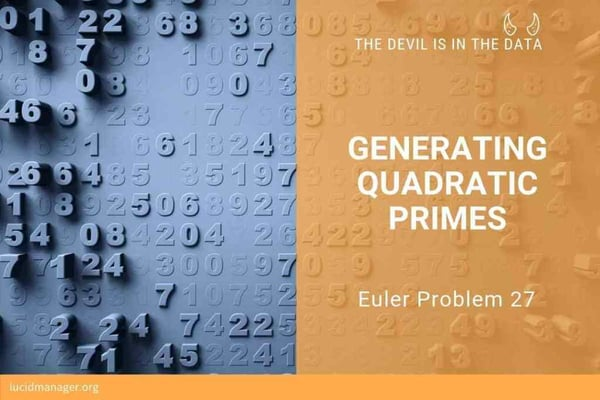Related Tags

euler problemEducative Answers Team

Prime numbers are vital to mathematics and economics all around the world, but they cannot be generated through an algorithm. Scientists and mathematicians are always trying to come up with formulas to generate prime numbers. Euler problem 27 deals with Quadratic Primes. Let’s have a look at the problem below.

## Euler Problem

Euler discovered the remarkable quadratic formula:

n2+n+41

It turns out that this formula will produce 40 primes for consecutive integer values, 0≤n≤39. However, this is not true when n=40, 402 +40+41= 40(40+1)+41 is divisible by 41, and certainly, when n=41, 412+41+41 is clearly divisible by 41.

Then, an incredible formula (n2−79n+1601) was discovered that produces 80 primes for the consecutive values, 0≤n≤79. The product of the coefficients −79 and 1601 is −126479.

n2+an+b, where |a|<1000 and |b|≤1000 where |n| is the modulus/absolute value of n, e.g., |11|=11 and |−4|=4

Find the product of the coefficients, a and b, for the quadratic expression that produces the maximum number of primes for consecutive values of n, starting with n=0.We need to find the values of a and b in the equation n2+an+b for a specific value n, which returns the product of the highest values of primes for a given n. The only way to solve this problem is through the brute force method. Brute force refers to trying each and every combination and checking whether it solves your problem. This kind of solution takes time to run for large values. Let’s look at a sample code below.

## Code

#include <iostream>
bool is_Prime(int x)
{
// invalid input
if (x <= 1)
return false;

// process all potential divisors
for(int i = 2; i <= x / 2; i++) {
if(x % i == 0) {
return false;
}
}

// no divisor found, therfore it's a prime number
return true;
}

int main()
{
// upper and lower limit
// change the value of limit according to your value
int value = 42;
// make sure it's a positive number
if (value < 0)
value = -value;

// number of generated primes of the best sequence
unsigned int count = 0;
// its coefficients
int value_a = 0;
int value_b = 0;

// Apply brute-force
for (int a = -value; a <= value; a++)
for (int b = -value; b <= value; b++)
{
// count of consecutive prime numbers
unsigned int l = 0;
while (is_Prime(l * l + a * l + b))
l++;

if (count < l)
{
count = l;
value_a = a;
value_b = b;
}
}

std::cout <<"Product value = " << (value_a * value_b) << std::endl;

std::cout << "a: "<< value_a << " " << "b: "<< value_b << std::endl;
return 0;
}


RELATED TAGS

euler problem